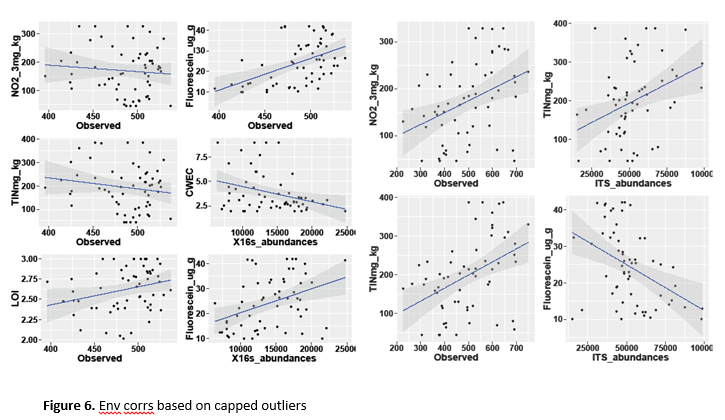## Visualising multiple (linear) regression lines in the same graph

Hello,

I have a dataset that I would like to visualize in VA in the following way:

I want a regression line per block of data (by chemical compound and by experiment number).

My regression is: Concentration=Intercept+Slope*Area.

What I need is a big graph that will be split into little squares and every square should contain a linear regression per block of data.

Here is an approximate image of what it should look like (please ignore values and names in the graphs):

COMPOUND 1                                                                 COMPOUND 2

Experiment 1                         Experiment 2                          Experiment 1                     Experiment 2Is it possible to do something like this in VA? I would also like to know how to automatically print the regression equation on each graph (or underneath it).

1 ACCEPTED SOLUTION

Accepted Solutions

## Re: Visualising multiple (linear) regression lines in the same graph

Elle, this may be possible to build in custom graph builder. Looks like you would have to start with a scatter plot and add a line plot and a band plot. The difficult thing is that you will have to have the upper and lower confidence bands and the equation value calculated and part of the data before starting.
Having the equation in the graph would require you to have a column called 'Equation' with the text "y=mx+b" for each applicable row., but it could be done.